# Product of 2-sphere and circle

## Definition

This topological space is defined as the Cartesian product, equipped with the product topology, of the 2-sphere$S^2$ and the circle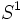$S^1$. It is denoted$S^2 \times S^1$ or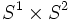$S^1 \times S^2$.

## Topological space properties

Property Satisfied? Is the property a homotopy-invariant property of topological spaces? Explanation Corollary properties satisfied/dissatisfied
manifold Yes No product of manifolds is manifold satisfies: metrizable space, second-countable space, and all the separation axioms down from perfectly normal space and monotonically normal space, including normal, completely regular, regular, Hausdorff, etc.
path-connected space Yes Yes path-connectedness is product-closed satisfies: connected space, connected manifold, homogeneous space (via connected manifold, see connected manifold implies homogeneous)
simply connected space No Yes Product of two spaces, one of which (the circle) is not simply connected. dissatisfies: simply connected manifold
rationally acyclic space No Yes Product of spaces neither of which is rationally acyclic. dissatisfies: acyclic space, weakly contractible space, contractible space
space with Euler characteristic zero Yes Yes Product of two spaces, one of which (the circle) has Euler characteristic zero. Note that Euler characteristic of product is product of Euler characteristics
space with Euler characteristic one No Yes See above, the Euler characteristic is zero
compact space Yes No Product of compact spaces, see Tychonoff's theorem satisfies: compact manifold, compact polyhedron, polyhedron (via compact manifold), compact Hausdorff space, and all properties weaker than compactness

## Algebraic topology

### Homology groups

Further information: homology of product of spheres

The homology groups with coefficients in integers are as follows: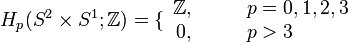$H_p(S^2 \times S^1;\mathbb{Z}) = \lbrace\begin{array}{rl} \mathbb{Z}, & \qquad p = 0,1,2,3 \\ 0, & \qquad p > 3 \\\end{array}$

The homology groups with coefficients in any module$M$ over a ring are as follows:$H_p(S^2 \times S^1;M) = \lbrace\begin{array}{rl} M, & \qquad p = 0,1,2,3 \\ 0, & \qquad p > 3 \\\end{array}$

### Cohomology groups

Further information: cohomology of product of spheres

The cohomology groups with coefficients in integers are as follows: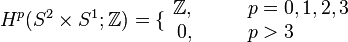$H^p(S^2 \times S^1;\mathbb{Z}) = \lbrace\begin{array}{rl} \mathbb{Z}, & \qquad p = 0,1,2,3 \\ 0, & \qquad p > 3 \\\end{array}$

The cohomology groups with coefficients in any module$M$ over a ring are as follows: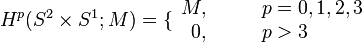$H^p(S^2 \times S^1;M) = \lbrace\begin{array}{rl} M, & \qquad p = 0,1,2,3 \\ 0, & \qquad p > 3 \\\end{array}$

### Homology-based invariants

Invariant General description Description of value for$S^2 \times S^1$ Comment
Betti numbers The$k^{th}$ Betti number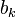$b_k$ is the rank of the torsion-free part of the$k^{th}$ homology group.$b_0 = b_1 = b_2 = b_3 = 1$, all higher$b_k$s are zero.
Poincare polynomial Generating polynomial for Betti numbers, i.e., the polynomial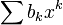$\sum b_kx^k$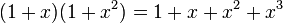$(1 + x)(1 + x^2) = 1 + x + x^2 + x^3$ See also Poincare polynomial of product is product of Poincare polynomials. The Poincare polynomial for$S^{m_1} \times S^{m_2} \times \dots \times S^{m_r}$ is$\prod_{i=1}^r (1 + x^{m_i})$
Euler characteristic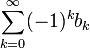$\sum_{k=0}^\infty (-1)^k b_k$ 0 Follows from Euler characteristic of product is product of Euler characteristics. In particular, the Euler characteristic of a product space is zero if any of the factor spaces has Euler characteristic zero.